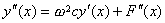[格林函数]  在区间[a,b]上，考虑微分方程

Ly+Φ(x)=0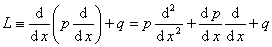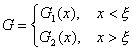(i)  函数G1G2在它们的定义区间上满足LG=0,即当x<ξ,LG1=0;x>ξ时，LG2=0

(ii)  函数G满足边界条件，即G1满足在x=a的边界条件，G2满足在x=b的边界条件。

(iii)  函数Gx=ξ连续，即G1(ξ)=G2(ξ)

(iv)  G的导数以x=ξ为一不连续点，其跳跃是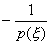，即

`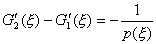`

`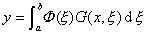(2)`

`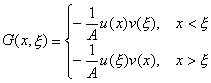(3)`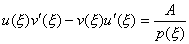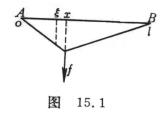[线性积分方程的一个典型实例]  考虑一条长为l的有弹性的弦，假定在平衡位置时，弦的位置在Ox轴的线段Ol上。在点x施加单位力，于是弦的每一点得到一个离差，在点x处所产生的离差以G(x,x)表示（图15.1）。函数G(x,x)为两点（xx）函数，在点x施加外力，在点x计量离差，称G为影响函数。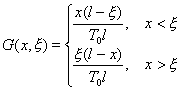G(x,x )=G(x , x)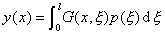设在某个力的作用下，弦成已知形状y=y(x),求定力分布强度p(x ),就得到含未知函数p(x )的第一类Fr积分方程(1)

设作用力随时间t改变，且在点x的强度是

p(x )sinw t                   (w >0)

y=y(x)sinw t

r (x )为弦在点x的线性密度，则在时刻t,xx +Dx 之间的小弦段除受力p(x)sinw tDx 的作用外，还受惯性力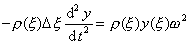sinw tDx

`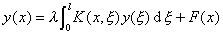(2)`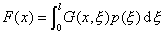K(x,x )=G(x,x )r (x ),           l =w 2

F(0)=F(l)=0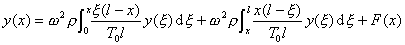`(3)`(3)式微分两次就得到